# Cotorsion-free group

(diff) ← Older revision | Latest revision (diff) | Newer revision → (diff)
An Abelian group is cotorsion-free if it does not contain any non-zero cotorsion group. More explicitly, this means that it contains no subgroup isomorphic to the additive groupof rational numbers, or to the additive group of-adic integers for any prime, and contains no cyclic group of prime order (thus, it is torsion-free). Equivalently, an Abelian groupis cotorsion-free if and only if, where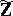denotes the completion of the group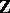of integers in its-adic topology. Cotorsion-free rings are rings (cf. Ring) whose additive groups are cotorsion-free Abelian groups. These rings play a distinguished role in the realization of rings as endomorphism rings of Abelian groups.
The celebrated theorem of Corner [a1] states that any countable cotorsion-free ringwith identity is isomorphic to the endomorphism ring of a countable reduced torsion-free Abelian group. Moreover, if the additive group ofhas finite rank, thencan be chosen to have rank at most. Corner's theorem has been generalized in various directions, notably to rings of arbitrary cardinality. The best result is due to R. Göbel and S. Shelah [a3]: Letbe a cotorsion-free ring with identity anda cardinal number such that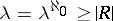. There are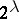cotorsion-free groups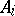of cardinalitywhose endomorphism rings are isomorphic tosuch that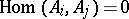for.
These results have several applications to the construction of counterexamples for torsion-free Abelian groups, e.g. to Kaplansky's test problems. Another consequence is the existence of arbitrarily large indecomposable Abelian groups. There is a topological version of the above theorem. If the endomorphism ring of an Abelian groupis equipped with the finite topology, then it becomes a complete Hausdorff topological ring (cf. also Hausdorff space). The Corner–Göbel theorem [a2] states that a topological ringwith identity is isomorphic to the finitely topologized endomorphism ring of a cotorsion-free Abelian groupif and only ifis complete Hausdorff in its topology and admits a base of neighbourhoods ofconsisting of left idealssuch thatis cotorsion-free (the endomorphisms act on the left). The Göbel–Shelah theorem [a3] generalizes to cotorsion-free algebras over commutative domains. The proof relies on the most useful black box principle.